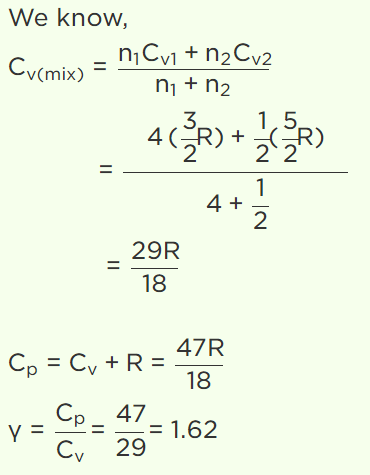## Kinetic Theory Questions and Answers Part-21

1. 5 moles of oxygen is heated at constant volume from 10°C to 20°C. The change in the internal energy of the gas is (the gram molecular specific heat of oxygen at constant pressure, $C_{P}$ = 8 cal/mole °C and R = 8.3 cal/mole °C)
a) 200 cal
b) 300 cal
c) 100 cal
d) None of these

Explanation: 300 cal

2. What is the value of $\frac{R}{C_{P}}$ for diatomic gas
a) 3/4
b) 3/5
c) 2/7
d) 5/7

Explanation: The value of $\frac{R}{C_{P}}$ for diatomic gas is 2/7

3. The degrees of freedom of a stationary rigid body about its axis will be
a) One
b) Two
c) Three
d) Four

Explanation: The degrees of freedom of a stationary rigid body about its axis will be 3

4. A gaseous mixture consists of 16g of helium and 16g of oxygen. The ratio $\frac{C_{P}}{C_{V}}$ of the mixture is
a) 1.4
b) 1.54
c) 1.59
d) 1.62

Explanation:5. The pressure exerted by the gas on the walls of the container because
a) It loses kinetic energy
b) It sticks with the walls
c) On collision with the walls there is a change in momentum
d) It is accelerated towards the walls

Explanation: The pressure exerted by the gas on the walls of the container because on collision with the walls there is a change in momentum

6. Gas at a pressure $P_{0}$ in contained is a vessel. If the masses of all the molecules are halved and their speeds are doubled, the resulting pressure P will be equal to
a) $4P_{0}$
b) $2P_{0}$
c) $P_{0}$
d) $\frac{P_{0}}{2}$

Explanation:7. A box contains n molecules of a gas. How will the pressure of the gas be effected, if the number of molecules is made 2n
a) Pressure will decrease
b) Pressure will remain unchanged
c) Pressure will be doubled
d) Pressure will become three times

Explanation: A box contains n molecules of a gas. if the number of molecules is made 2n then pressure will be doubled

8. The relation between the gas pressure P and average kinetic energy per unit volume E is
a) $P=\frac{1}{2}E$
b) P = E
c) $P=\frac{3}{2}E$
d) $P=\frac{2}{3}E$

Explanation: The relation between the gas pressure P and average kinetic energy per unit volume E is $P=\frac{2}{3}E$

9. A cylinder of capacity 20 litres is filled with $H_{2}$  gas. The total average kinetic energy of translatory motion of its molecules is $1.5\times 10^{5} J$  . The pressure of hydrogen in the cylinder is
a) $2\times 10^{6} N\diagup m^{2}$
b) $3\times 10^{6} N\diagup m^{2}$
c) $4\times 10^{6} N\diagup m^{2}$
d) $5\times 10^{6} N\diagup m^{2}$10. The root mean square speed of hydrogen molecules of an ideal hydrogen gas kept in a gas chamber at 0°C is 3180 metres/second. The pressure on the hydrogen gas is (Density of hydrogen gas is $8.99\times 10^{-2} kg\diagup m^{3}$   , 1 atmosphere = $1.01\times 10^{5} N\diagup m^{2}$   )